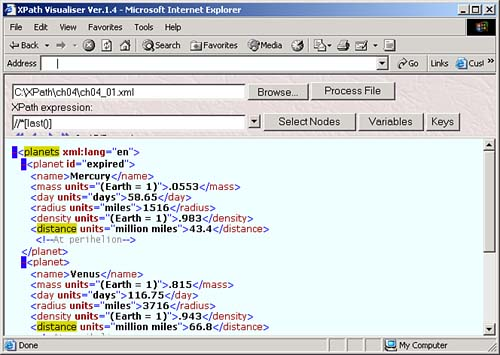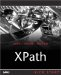The XPath Node-Set Operators and Functions

XPath does have a few operators that are designed to work on node-sets . In Chapter 3, we saw that the operator computes the union of its operands, which must be node-sets. Here's an example of an XSLT template that uses this operator, which we saw in Chapter 3:

<xsl:template match="name  day">     .     .     . </xsl:template>

Also, as you know, the / and // operators create location paths, and you must use these operators with expressions that evaluate to node-sets. You've already had plenty of practice with these operators.

Here are the XPath functions that work on node-sets:

• count( node-set ) This function returns the number of nodes in node-set .

• id(string ID ) This function returns a node-set of the element whose ID matches the string passed to the function, or an empty node-set if no element matches.

• last() This function returns the number of nodes in a node-set that contains the context nodes and all its siblings.

• local-name( node-set ) This function returns the local name of the first node in the node-set.

• name( node-set ) This function returns the qualified name of the first node in the node-set.

• namespace-uri( node-set ) This function returns the URI of the namespace of the first node in the node-set.

• position() This function returns the position of the context node in the context node-set, starting with 1.

We'll see these functions in more detail next .

The count Function

You use the count function to count the number of nodes in a node-set. All you have to do is to pass the node-set to this function, and it returns the number of nodes as a number.

You can see an example in the XPath Visualiser in Figure 4.10, where we're counting the number of <planet> elements.

Figure 4.10. Using the count function.Here's an example that we saw earlier in this chapter where we used the expression sum(//mass) div count(//mass) , which uses the count function to determine the average planetary mass:

<?xml version="1.0" encoding="UTF-8"?> <xsl:stylesheet version="1.1" xmlns:xsl="http://www.w3.org/1999/XSL/Transform"> <xsl:output method="xml"/> <xsl:template match="planets">     <HTML>         <BODY>             The average planetary mass is:  <xsl:value-of select="sum(//mass) div count(//mass)"/>  </BODY>     </HTML> </xsl:template> </xsl:stylesheet>

And here's the resulting document:

<?xml version="1.0" encoding="UTF-8"?> <HTML>     <BODY>         The average planetary mass is: 0.6234333333333334     </BODY> </HTML>

The id Function

The id function returns a node-set where all the nodes have the same ID value as that passed to this function.

Here's an XSLT template that matches all elements that have the ID " expired ":

<xsl:template match = "//*[id('expired')]">     <xsl:value-of select="."/> </xsl:template>

To make this work, XPath and XSLT processors usually require that you declare ID values as such in a DTD or XML schema. For example, if you wanted to create a DTD for ch04_01.xml that supported an id attribute of XML type ID, and set that attribute to "expired" in the <planet> element for Mercury, here's what that might look like:

<?xml version="1.0" encoding="UTF-8"?> <!DOCTYPE planets [ <!ELEMENT planet (name, mass, radius,day)> <!ELEMENT name (#PCDATA)> <!ELEMENT mass (#PCDATA)> <!ELEMENT radius (#PCDATA)> <!ELEMENT day (#PCDATA)>  <!ATTLIST planet   id ID #REQUIRED>   ]>  <planets>  <planet id='expired'>  <name>Mercury</name>         <mass units="(Earth = 1)">.0553</mass>         <day units="days">54.65</day>         <radius units="miles">1516</radius>         <density units="(Earth = 1)">.983</density>         <distance units="million miles">43.4</distance><!--At perihelion-->     </planet>         .         .         .

BE CAREFUL WHEN MATCHING BY ID

Some XPath processors and some XSLT processors won't match by ID because they won't read DTDs or XML schema.

The last Function

The last function returns the number of nodes in the node-set that contains the context node and its siblings, so its value is equal to the position of the last node. You can see an example in the XPath Visualiser in Figure 4.11 where in this case we're selecting the last element in each sibling group with the location path //*[last()] .

Figure 4.11. Using the last function.Here's an XSLT example. In this case, we'll use the position and last functions to indicate the order of the planets, as you can see in ch04_05.xsl in Listing 4.4.

Listing 4.4 Using the last Function ( ch04_05.xsl )
<?xml version="1.0" encoding="UTF-8"?> <xsl:stylesheet version="1.1" xmlns:xsl="http://www.w3.org/1999/XSL/Transform"> <xml:output method="xml"/> <xsl:template match="planets"> <document>     <planets>         <xsl:apply-templates select="planet"/>     </planets> </document> </xsl:template>  <xsl:template match="planet">   <xsl:value-of select="name"/> is planet   <xsl:text> </xsl:text>   <xsl:value-of select="position()"/> of   <xsl:text> </xsl:text>   <xsl:value-of select="last()"/>   <BR/>   </xsl:template>  </xsl:stylesheet>

Here's the result"Mercury is planet 1 of 3", "Venus is planet 2 of 3", and so on:

<?xml version="1.0" encoding="UTF-8"?> <document>     <planets>  Mercury is planet 1 of 3   <BR/>   Venus is planet 2 of 3   <BR/>   Earth is planet 3 of 3   <BR/>  </planets> </document>

The local-name Function

You can use the local-name function to return the local (that is, unqualified) name of a node. You pass this function a node-set and it returns the local name of the first name in the node-set. If the node-set is empty, it returns the local name of the context node.

You can see an example in the XPath Visualiser in Figure 4.12, where we're finding all <radius> elements using the location path //*[local-name()="radius"] .

Figure 4.12. Using the local-name function.The name Function

The name function is like the local-name function, except that it returns the fully qualified name of the node, including any namespace. For example, if you set the document element's namespace to the fictitious URI "http://www.XPathCorp.com" like this:

<?xml version="1.0" encoding="UTF-8"?>  <planets xmlns:ns="http://www.XPathCorp.com" xml:lang="en">  <ns:planet>         <ns:name>Mercury</ns:name>         <ns:mass units="(Earth = 1)">.0553</ns:mass>         <ns:day units="days">58.65</ns:day>         .         .         .

Then all the child elements would have the namespace "http://www.XPathCorp.com". For example, the expression name(//planet) returns the full name of the first <planet> element. Different XPath processors have different ways of doing thissome will return "http://www.XPathCorp.com:planet", and some will return programming objects that you need to decipher with programming code.

The namespace-uri Function

You can use the namespace-uri function in XPath 1.0 to get a string containing the URI of the namespace in a node's name. This is the URI in a namespace declaration as set with the xmlns or xmlns: prefix attributes. You can only use this function on elements or attributes in node-sets.

For example, you might add a namespace, " pickle ", to ch04_01.xml :

<?xml version="1.0" encoding="UTF-8"?>  <pickle:planets xmlns:pickle="http://XPathCorp.com">   <pickle:planet>   <pickle:name>Mercury</pickle:name>   <pickle:mass units="(Earth = 1)">.0553</pickle:mass>   <pickle:day units="days">54.65</pickle:day>   <pickle:radius units="miles">1516</pickle:radius>   <pickle:density units="(Earth = 1)">.983</pickle:density>   <pickle:distance units="million miles">43.4</pickle:distance>   <!--At perihelion-->   </pickle:planet>   <pickle:planet>   <pickle:name>Venus</pickle:name>   <pickle:mass units="(Earth = 1)">.815</pickle:mass>   <pickle:day units="days">116.75</pickle:day>   <pickle:radius units="miles">3716</pickle:radius>   <pickle:density units="(Earth = 1)">.943</pickle:density>   <pickle:distance units="million miles">66.8</pickle:distance>   <!--At perihelion-->   </pickle:planet>  .         .         .

Then you could find the URI of this namespace in a stylesheet using namespace-uri :

<?xml version="1.0" encoding="UTF-8"?> <xsl:stylesheet version="1.1" xmlns:xsl="http://www.w3.org/1999/XSL/Transform" xmlns:pickle="http://www.XPathCorp.com"> <xsl:template match="/planets">  <xsl:value-of select="namespace-uri()"/>  </xsl:template>         .         .         .

Here's what you'd see as the result:

<?xml version="1.0" encoding="UTF-8"?> http://XPathCorp.com

The position Function

We've already seen the position function, which returns the position of the context node in the node-set that makes up the current context. This function is a favorite, and we've put it to work throughout the book already. Here's an example we've seen earlier in this chapter that uses the position function to display the location of each planet:

<?xml version="1.0" encoding="UTF-8"?> <xsl:stylesheet version="1.1" xmlns:xsl="http://www.w3.org/1999/XSL/Transform"> <xml:output method="xml"/> <xsl:template match="planets"> <document>     <planets>         <xsl:apply-templates select="planet"/>     </planets> </document> </xsl:template>  <xsl:template match="planet">   <xsl:value-of select="name"/> is planet   <xsl:text> </xsl:text>   <xsl:value-of select="position()"/> of   <xsl:text> </xsl:text>   <xsl:value-of select="last()"/>   <BR/>   </xsl:template>  </xsl:stylesheet>

And here's the result:

<?xml version="1.0" encoding="UTF-8"?> <document>     <planets>  Mercury is planet 1 of 3   <BR/>   Venus is planet 2 of 3   <BR/>   Earth is planet 3 of 3   <BR/>  </planets> </document>

That completes the XPath 1.0 node-set functions, as well as our discussion on the operators and functions available in XPath 1.0. You can see the support for XPath 1.0 functions in Internet Explorer in Table 4.1, listed by XML processor, MSXML, version.

Table 4.1. The XPath 1.0 Functions in Internet Explorer

FUNCTION

MSXML2

MSXML3

MSXML4

MSXML.NET

boolean

X

X

X

ceiling

X

X

X

concat

X

X

X

contains

X

X

X

count

X

X

X

false

X

X

X

floor

X

X

X

id

X

X

X

X

lang

X

X

X

last

X

X

X

X

local-name

X

X

X

name

X

X

X

namespace-uri

X

X

X

normalize-space

X

X

X

not

X

X

X

number

X

X

X

position

X

X

X

round

X

X

X

starts-with

X

X

X

string

X

X

X

string-length

X

X

X

substring

X

X

X

substring-after

X

X

X

substring-before

X

X

X

sum

X

X

X

translate

X

X

X

true

X

X

X

That wraps up this chapter, where we've seen the available XPath 1.0 operators and functions. You'll see these operators and functions in XPath predicates all the time. In the next chapter, we'll take a look at working with XSLT with XPath.XPath Kick Start: Navigating XML with XPath 1.0 and 2.0
ISBN: 0672324113
EAN: 2147483647
Year: 2002
Pages: 131

Similar book on Amazon